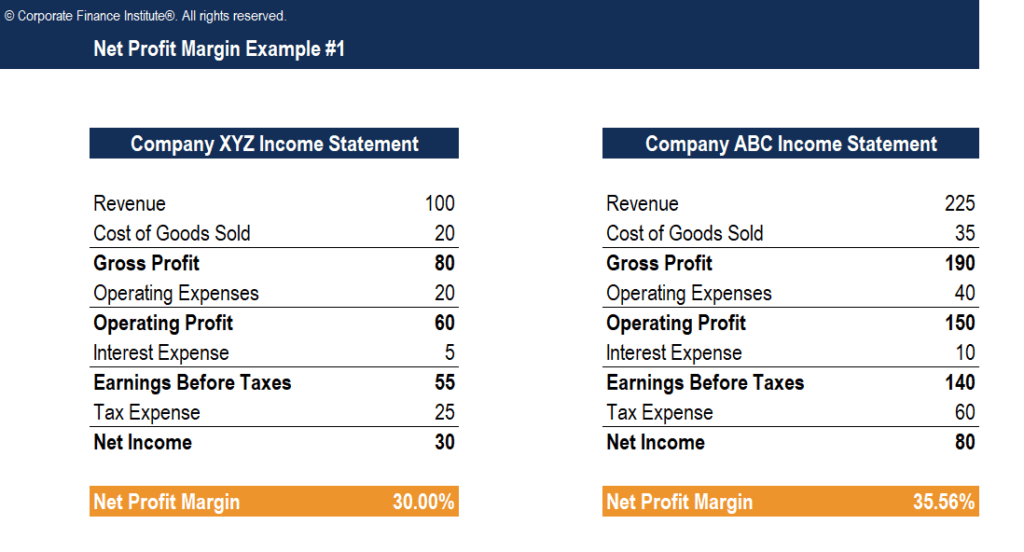# Net Profit Margin Template

## Net Profit Margin Template

This Net Profit Margin template will show you how to calculate the net profit margin using a company’s income statement line items.

Here is a screenshot of the template:### What is Net Profit Margin?

Net Profit Margin (also known as “Profit Margin” or “Net Profit Margin Ratio”) is a financial ratio used to calculate the percentage of profit a company produces from its total revenue. It measures the amount of net profit a company obtains per dollar of revenue gained. The net profit margin is equal to net profit (also known as net income) divided by total revenue, expressed as a percentage.

### Net Profit Margin Formula

Net Profit margin = Net Profit  ⁄  Total revenue x 100

Net profit is calculated by deducting all company expenses from its total revenue. The result of the profit margin calculation is a percentage – for example, a 10% profit margin means for each \$1 of revenue the company earns \$0.10 in net profit. Revenue represents the total sales of the company in a period.

### More Free Templates

For more resources, check out our business templates library to download numerous free Excel modeling, PowerPoint presentation and Word document templates.

• Excel Modeling Templates
• PowerPoint Presentation Templates
• Transaction Document Templates

### Financial Analyst Certification

Become a certified Financial Modeling and Valuation Analyst (FMVA)® by completing CFI’s online financial modeling classes and training program!